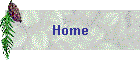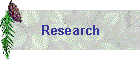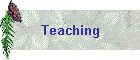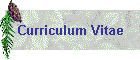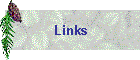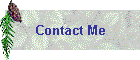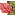Course:Numerical methods for partial differential equations and their applications in biology.References: (1) Finite Difference Method for Ordinary and Partial Differential Equations, Steady-State and Time-Dependent Problem by Randall J. LeVeque, SIAM, 2007 (2) A first Course in the Numerical Analysis of Differential Equations by Arieh Iserles, Cambridge Textx in Applied mathematics, 1996 (3) Numerical Solution of Partial Differential equations, Finite Difference Methods, by G. D. Smith, third edition, Oxford Applied Mathematics and Computing Science Series, 1985 (4) Understanding and Implementing the Finite Element Method, by Mark S. Gockenbach, SIAM, 2006 (5) Numerical Methods for Conservation Laws by Randall J. LeVeque, Birkhauser, 1992 (6) Level set methods and dynamic implicit surfaces by Stanley Osher and Ronald P. Fedkiw, Springer, 2003 (7) Computer Methods for Ordinary Differential Equations and Differential-Algebraic Equations by Uri M. Ascher and Linda R. Petzold, SIAM, 1998Tentative Schedule: updated regularly

 Tuesday: MBI Lecture Hall Thursday: MBI Lecture Hall Jan 5 Overview of Numerical Methods for PDEs, Method of lines (MOL) for time-dependent PDEs, Ref: (1) Chapter 9.2 (p.184) ODE: explicit, accuracy, stability, convergene Runge-Kutta, RK45, SSP RK Ref: (2) & (7) Chapter 1-5 Jan 7 ODE: implicit scheme, consistency, stability, convergence, ode15s Ref: (2) & (7) Chapter 1-5 Jan 12 Parabolic equation: diffusion, accuracy, stability Ref: (1) Chapter 9 and (3) Chapter 2-3 Jan 14 Parabolic equation: diffusion-reaction Ref: (1) Chapter 9 and (3) Chapter 2-3 Jan 19 parabolic equation: stiffness Ref: (1) Chapter 9 and (3) Chapter 2-3 Jan 21 elliptic equation, finite difference, linear, nonlinear, iterative scheme Ref: (3) Chapter 5 and (4) Chapter 10-12 Jan 26 elliptic equation, multigrid Ref: (3) Chapter 13 Jan 28 elliptic & parabolic equation, finite element, (matlab, comsol) Ref: (3) Chapter 5 and(4) Chapter 4 Feb 2 hyperbolic equation, theory Ref: (5) cahpter 1-6 Feb 4 hyperbolic equation, numerical methods Ref: (5) cahpter 1-6 Feb 9 hyperbolic equation, ENO, WENO, and DG Ref: (5) Chapter 10-18 Feb 11 introduction to free interface problems and numerical techniques mapping methods in 1D and 2D (conformal mapping) Feb 16 guest lecture: Monte-Carlo methods Feb 18 guest lecture: SODEs Feb 22 MBI workshop Feb 24 MBI workshop Mar 2 Level Set Method Ref: (6) Chapter 1-8 Mar 4 Level Set Method Mar 9 IMA conference Mar 11 IMA conference Mar 15 MBI workshop Mar 17 MBI workshop